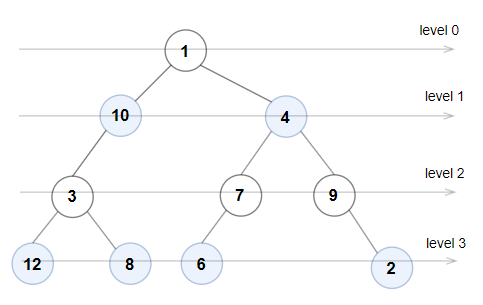# Leetcode 1609 - Even Odd Tree

Note:

• Classic level-order traversal problem.
• Use queue.

Question:

A binary tree is named Even-Odd if it meets the following conditions:

• The root of the binary tree is at level index 0, its children are at level index 1, their children are at level index 2, etc.
• For every even-indexed level, all nodes at the level have odd integer values in strictly increasing order (from left to right).
• For every odd-indexed level, all nodes at the level have even integer values in strictly decreasing order (from left to right).

Given the root of a binary tree, return true if the binary tree is Even-Odd, otherwise return false.

Example:Code: